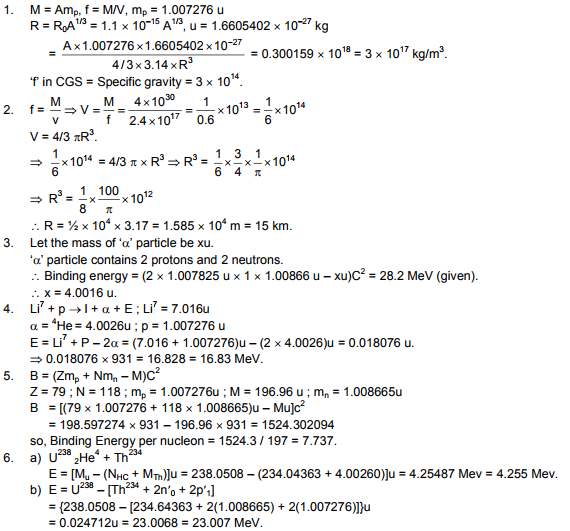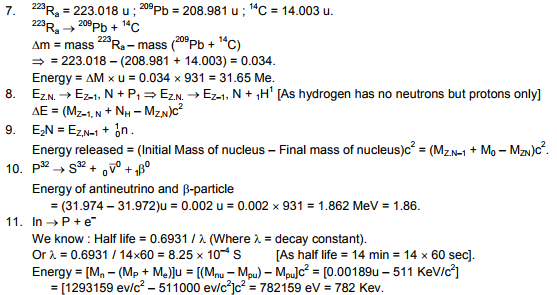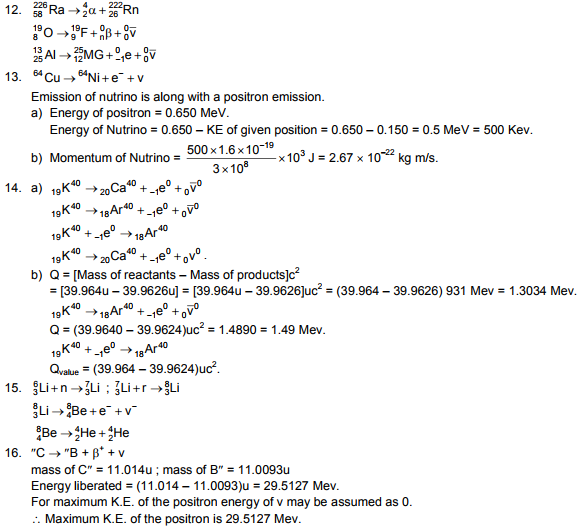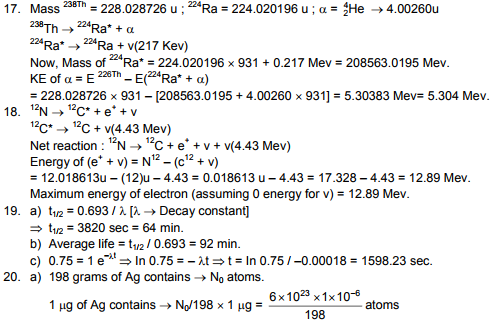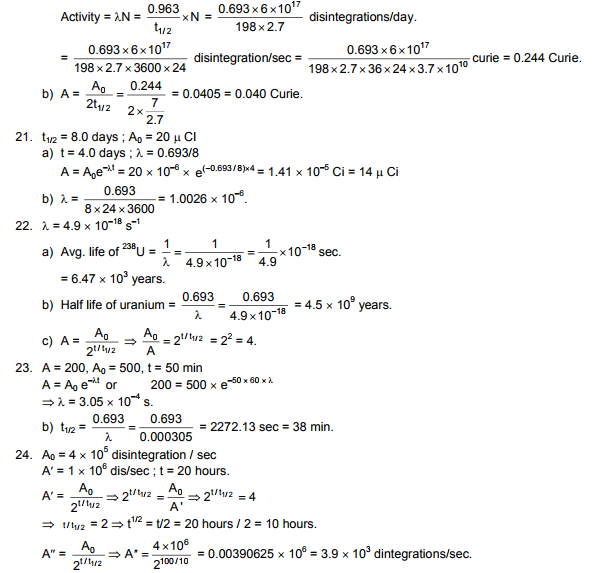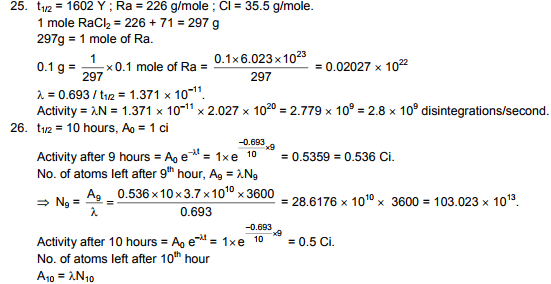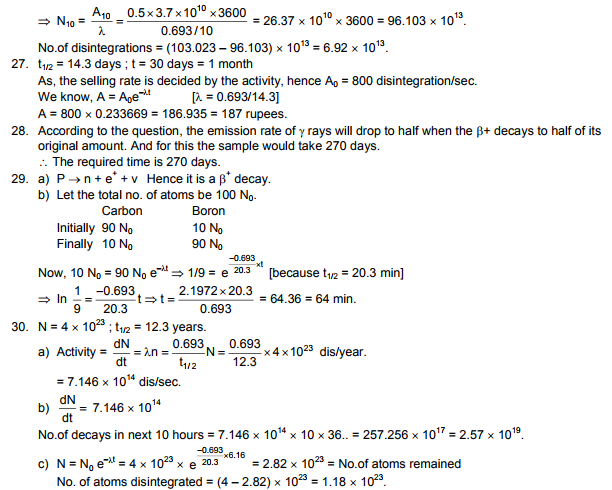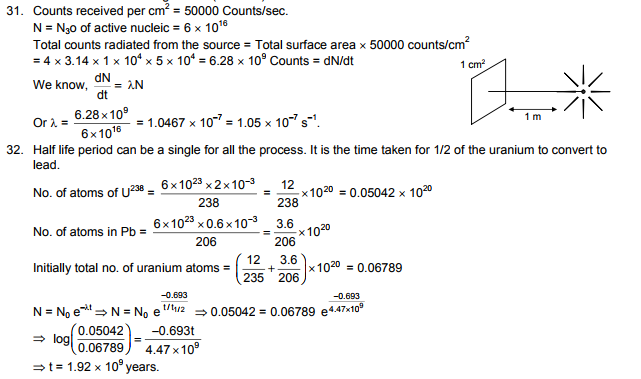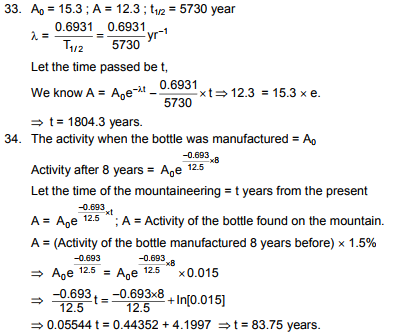# HC Verma Solutions Vol 2 Chapter 24 The Nucleus

HC Verma Solutions Vol 2 Chapter 24 The Nucleus is one of the most useful study materials for students especially those who are in 12th standard studying physics or those who are making use of the HC Verma book part 2. The solutions contain answers that are described and formulated in the best way possible for students to clearly understand​ and learn the topics as well as the exercises discussed in the chapter. The solutions also cover questions that are frequent in exams like JEE. The solutions are also designed in such a manner in order to give students better learning experience and solve questions easily. Some of the problems given here include:

• Finding the binding energy of protons and the neutrons.
• There are questions related to half-life period and atoms of uranium and the counts radiated from the source, the number of atoms disintegrated and the number of decays.
• Students will also solve questions based on energy liberated and momentum of positron and neutrino particles and many more.

These solutions can be a great study material for daily practice and a serious reference tool while preparing for competitive exams. HC Verma solutions are highly recommended and will enhance students’ abilities to tackle any type of questions that may be asked in the exams.

## Key Topics Related To The Nucleus

• Properties of Nucleus
• Nuclear Forces
• Binding Energy
• Properties and Uses of Nuclear Radiation
• Nuclear Fission
• Uranium Fission Reactor
• Nuclear Fusion

## Important Questions In Chapter 24

1. When we separate nucleons of a nucleus from each other, there is an increase in the total mass. Can you explain where does this mass originate from?

2. During gamma decay, does the nucleus experience loss of mass?

3. An electron (or a positron) is generally released by a nucleus during beta decay. Do atoms that are usually left behind get oppositely charged when this occurs?

4. What happens to the binding energy of a nucleus when the mass number X is increased?

(a) the energy increases (b) It decreases (c) No change (d) It varies depending on the original value of X

5. What occurs in one average-life of nuclei?

(a) all the nuclei decay (b) half the active nuclei decay (c) less than half the active nuclei decay (d) more than half the active nuclei decay

## HC Verma Solutions Vol 2 The Nucleus Chapter 24